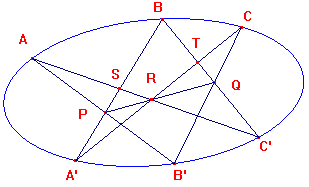Chasles Theorem

An alternative approach to Pascal's Theorem uses a beautifully simple result due to Chasles.

We begin with the dual of the cross-ratio of four points on a line, namely the cross-ratio of
four lines through a point. We refer to such a configuration as a p-pencil and the common
point as its vertex

lemma 1
If A,B,C,D are p-lines through a p-point P and L a p-line meeting
A,B,C,D in A,B,C,D respectively, then the cross-ratio (A,B,C,D)
is independent of the choice of L.

This allows us to make the

definition
If A,B,C,D are p-lines through a p-point P, the cross-ratio (A,B,C,D)
is the cross-ratio of the p-points where the p-lines meet any p-line L

Since the cross-ratio of points is a projective invariant, we immediately have

lemma 2
The cross-ratio of a p-pencil is a projective invariant

If we look at an embedding of a p-pencil and p-line L, we get a pencil on the embedding
plane with vertex P and a line L cutting the lines of the pencil at A,B,C,D. The cross-ratio
of the p-pencil is equal to (A,B,C,D), and may be denoted by (PA,PB,PC,PD), though it
depends only on the lines, and not on the points chosen.

lemma 3
If a line cuts the lines of a pencil with vertex P at the points A,B,C,D,
then (PA,PB,PC,PD) = sin(<APC)sin(<BPD)/sin(<BPC)sin(<APD).

 lemma 4 Suppose that we have five points P,P',Q,R,S on a circle. If P,P' lie on the same side of RS, then as signed angles, then sin(

Pascal's Theorem
If A,A',B'B',C,C' lie on a conic C and AB',A'B meet in P,
BC',B'C in Q and CA',C'A in R, then P,Q,R are collinear.proof
By Chasles' Theorem, (AA',AB',AC',AB) = (CA',CB',CC',CB).
As remarked above, these are equal to the cross-ratios of
the points which are intersections of the pencils with BA', BC'.
Thus (A',P,S,B) = (T,Q,C',B).
Now consider these cross-ratios as those of pencils through R.
(RA',RP,RA,RB) = (RC,RQ,RC',RB). As lines, RA'=RC, RA=RC',
so the pencils have three lines in common. Since their cross-
ratios are equal, the lines RP,RQ are identical, as required.